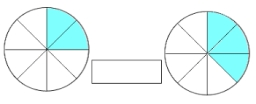# True or false?

Which of the following is true?

A. Three and three ninths plus seven and six-elevenths equal ten and eighty-seven ninety ninths.
B. two and three-eighths plus six and four-fifths equals eight and twelve fortieth
C. three and three sevenths plus four and two-thirds equals seven and two twenty ones.
D. four and five-sixths plus five sevenths equal nine and ten thirteenths

Result

A=

B=

C=

D=

### Step-by-step explanation:Did you find an error or inaccuracy? Feel free to write us. Thank you!

Showing 1 comment:
Math student

Tips for related online calculators
Need help calculating sum, simplifying, or multiplying fractions? Try our fraction calculator.
Need help with mixed numbers? Try our mixed-number calculator.